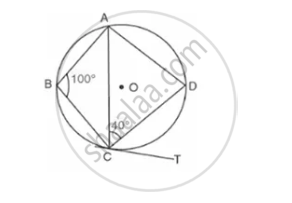Share

Books Shortlist

# In the Given Circle with Center O, ∠Abc=100°, ∠Acd=40° and Ct is Tangent to the Circle at C. Find ∠Adc and ∠Dct. - CBSE Class 10 - Mathematics

ConceptNumber of Tangents from a Point on a Circle

#### Question

In the given circle with center o, ∠ABC=100°, ∠ACD=40° and CT is tangent to the circle at C. find ∠ADC and ∠DCT.#### Solution

∠ ABC+∠ADC=180°   (Opposite angle of a cydic quadrilateral are supplementary)

⇒ 100°+∠ADC=180°

⇒ ∠ADC=80°

Now, in ΔACD,

∠ADC+∠CAD+∠ADC=180°

⇒40°+∠CAD+80°=180°

⇒∠CAD=180°-120°

⇒∠CAD=60°

"Now" ∠DCT=∠CAD ...........("angles in the alternate segment are equal")

∴ ∠DCT=60°

Is there an error in this question or solution?

#### Video TutorialsVIEW ALL 

Solution In the Given Circle with Center O, ∠Abc=100°, ∠Acd=40° and Ct is Tangent to the Circle at C. Find ∠Adc and ∠Dct. Concept: Number of Tangents from a Point on a Circle.
S